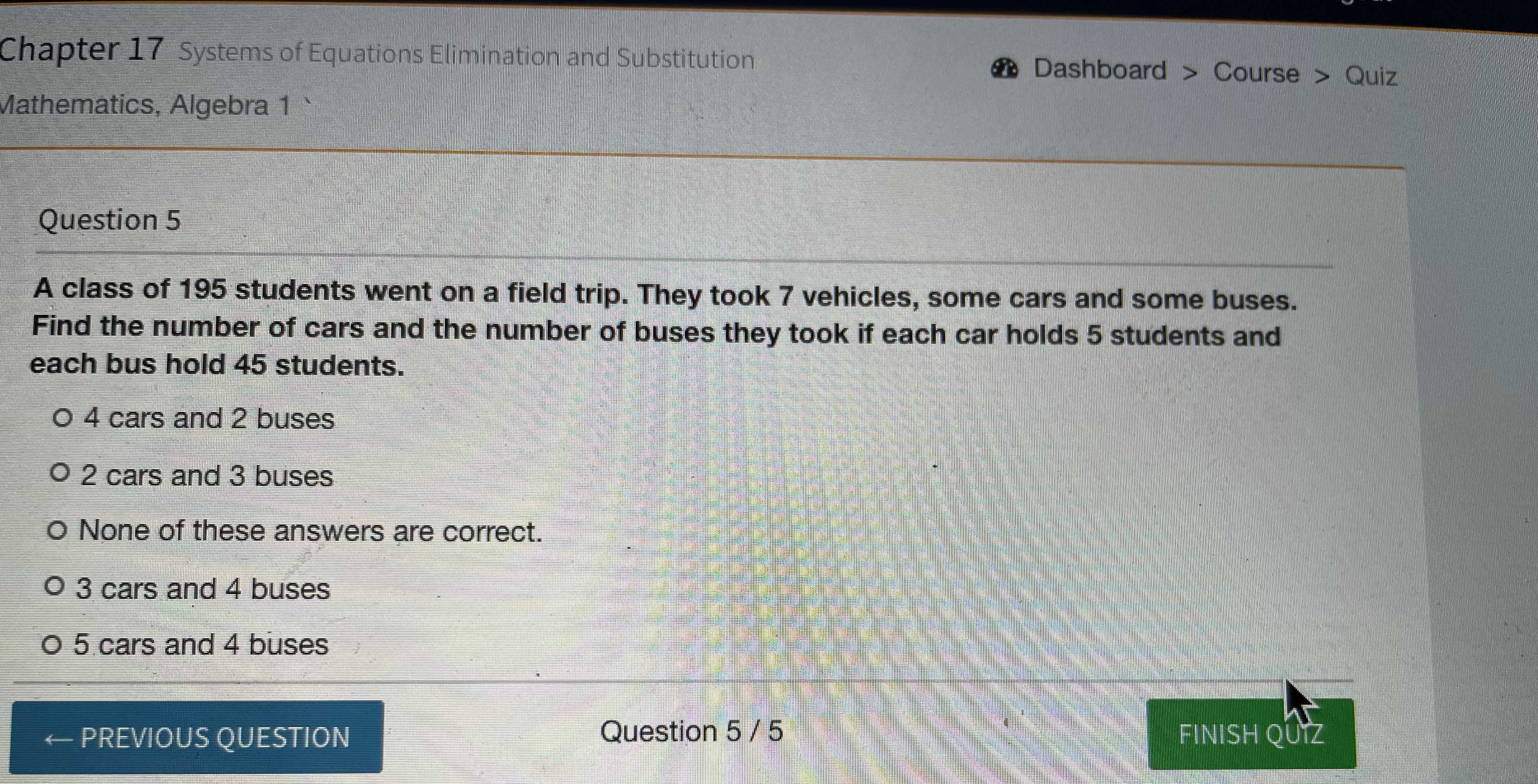### ¿Todavía tienes preguntas de matemáticas?

Pregunte a nuestros tutores expertos
Algebra
PreguntaA class of 195 students went on a field trip. They took 7 vehicles, some cars and some buses.

Find the number of cars and the number of buses they took if each car holds 5 students and each bus hold 45 students.

$$4$$ cars and $$2$$ buses

$$2$$ cars and $$3$$ buses

None of these answers are correct.

$$3$$ cars and $$4$$ buses

$$5$$ cars and $$4$$ buses